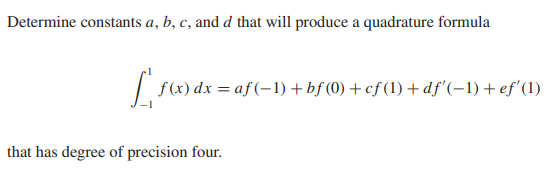# Question Determine constants $a, b, c$ , and d that will produce a quadrature formula $\int_{-1}^{1} f(x) d x=a f(-1)+b f(0)+c f(1)+d f^{\prime}(-1)+e f^{\prime}(1)$ that has degree of precision four.JFHWU1 The Asker · Other Mathematics
Determine constants   $a, b, c$  , and   d   that will produce a quadrature formula
$\int_{-1}^{1} f(x) d x=a f(-1)+b f(0)+c f(1)+d f^{\prime}(-1)+e f^{\prime}(1)$
that has degree of precision four.Transcribed Image Text: Determine constants $a, b, c$ , and d that will produce a quadrature formula $\int_{-1}^{1} f(x) d x=a f(-1)+b f(0)+c f(1)+d f^{\prime}(-1)+e f^{\prime}(1)$ that has degree of precision four.
More
Transcribed Image Text: Determine constants $a, b, c$ , and d that will produce a quadrature formula $\int_{-1}^{1} f(x) d x=a f(-1)+b f(0)+c f(1)+d f^{\prime}(-1)+e f^{\prime}(1)$ that has degree of precision four.